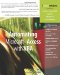# Using the Is Functions for Flawless Processing

 < Day Day Up >

### Using the Is Functions for Flawless Processing

All functions are prone to errors if you don't reference the right type of data. Most of these errors can be avoided by a simple check using one of VBA's many Is functions:

• IsArray Tests for an array

• IsDate Tests for a valid date value

• IsEmpty Determines whether variable has been initialized (by having stored a value)

• IsError Determines whether an expression is a valid error value

• IsMissing Determines whether an optional argument has been passed to a procedure

• IsNull Determines whether an expression contains a Null value

• IsNumeric Determines whether an expression can be evaluated as a valid number

• IsObject Indicates whether a variable contains a reference to an objectYou learn about arrays in Chapter 7, "Working with Arrays," (p. 105).

All the Is functions use the same simple form

` `

` Isfunction(value) `

where value represents the actual value, variable, argument, or expression being checked. Each function returns a Boolean value. If the value meets the condition being checked for the function returns True. Each function returns a False value if the condition isn't met. For instance, if the variable varValue is Null, the following function returns True:

` `

` IsNull(varValue) `

 < Day Day Up >Automating Microsoft Access with VBA
ISBN: 0789732440
EAN: 2147483647
Year: 2003
Pages: 186